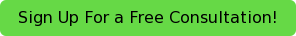# Tools to Cut Through the Quantitative Section of the GRE

By JeddThe right tools will allow you to cut through just about any problem on the GRE quantitative section.

# Many topics but a few key strategies

Probably the biggest challenge that students face when approaching the quantitative section on the GRE is the sheer range of topics, which cover numerous concepts up through early high school math. The upside is that not a single one of these topics is particularly complicated, and the way they are tested has more to do with reading carefully, using clues and cues embedded in the question and answer choices strategically, and pacing yourself according to your target score - slower for medium score and faster for high scores.

The topics themselves cover concepts in algebra, geometry (properties of shapes, formulas for calculating size), data analysis (chart and graph interpretation, statistics and probability), and number properties, (which is about having a deeper conceptual understanding of topics such as factoring, divisibility, exponents and roots, and positive and negative numbers).

## Some topics feel easier than others

Most students I’ve worked with are particularly rusty on exponent rules, some of the geometry concepts, probability, and combined rate problems simply because these topics don’t come up in most lines of work. For key formulas and relationships, I suggest that students begin by creating flashcards and then reinforce by working out concrete examples of each formula. An example of this might be calculating the value of [(2^3)^2] / (2^4) to reinforce the power and quotient exponent rules. Rather than immediately simplifying this to be (8^2)/16 = 4, I’d recommend that the student write it as:

(2*2*2)(2*2*2)  =   (2*2*2)(2*2*2)   =  2*2 = 4.

(2*2*2*2)             (2*2*2*2)

Reinforcing the intuitive logic of formulas and rules will help you to remember them, even when there are several dozen of them. (If you’re rusty on these exponent rules, just google them, and you’ll find lots of visually appealing layouts of these foundational rules.)

## A few key strategies

The most important global strategies include some that apply to any standardized test, such as knowing when to backsolve from the answer choices, pick your own numbers, or simplify algebraically, and some specific to the GRE, such as making the two terms in Quantitative Comparison problems resemble each other, skimming the Data Interpretation charts and figures without getting bogged down in the details, and flagging the most complex problems, such as “select all that apply,” to review at the end of the section rather than burning through extra minutes up front.

Getting a mid-range score, between the 40th and 60th percentiles, in the low 150s on each section, requires fluency with most of the math concepts and the skill of applying this knowledge accurately. Getting an upper range-score, at or above the 80th percentile, or 160 on each section, requires being able to engage in more complex inferences beyond application of basic knowledge. Here are examples of each.

## The difference between “medium” and “hard” questions

A mid-level quantitative comparison problem tells us that (x^2)y > 0, and x(y^2) < 0, and then it asks us whether x or y is larger. This problem requires us to understand how different kinds of multiplication result in positive versus negative numbers and infer from there: Squares are always positive, a positive times a positive is positive, and a positive times a negative is always negative. Since (x^2)y > 0, y must also be positive. Since x(y^2) < 0, x must be negative. We can conclude that  x<y, so the answer is B.

An upper-level quantitative comparison problem asks us to go just one step further. It tells us that the variable x is any integer greater than 1 and then asks us to compare the relative sizes of 3^(x+1) and 4^x. The former term has a smaller base but a bigger exponent. To solve this, there’s not a simple formula or rule that determines the answer. Instead, we need to consider at least a couple different values for x. When picking numbers, we always want to consider both small and large values, and if the problem permits, we want to consider whole numbers and fractions, as well as positive and negative numbers. This problem specified that x may only be positive integers greater than two, so picking x = 2 and 10 should be enough. If we set x equal to the minimum value of 2, the first term is 3^3 = 27, which is larger than 4^2 = 16. If we make x larger, we can use the onscreen calculator to find that 3^11 = 177,147, which is much smaller than 4^10 = 1,048,576. So the answer is D, which means “cannot be determined.”

# Keep your eye on the prize

An initial diagnostic test will be enough to tell you and your tutor which topics you need to review and which level of problems you should focus on first. Content coverage and a handful of key strategies will allow you cut through the jungle of GRE quant prep without too much trouble.

Are you feeling overwhelmed preparing for the GRE? Call in today to share where you are in the process, so we can make recommendations that are right for you.Do you want more tips on how best to prepare for test day?  Read some of our previous blogs on the GRE below!